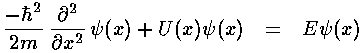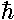## Falling p.2

In classical mechanics the primary aim is to solve Newton's equation, finding the position as a function of time:In quantum mechanics the primary aim is to solve the time independent Schrödinger's equation to find the probability amplitude (i.e., the wavefunction) as a function of position.Note again the huge shift between classical mechanics and quantum mechanics: what was the dependent variable in classical mechanics (x) has become the independent variable; what was the independent variable (t) has disappeared. Later we'll see how motion is possible if time plays no role. It turns out energy (E) is more measurable than motion, hence energy has replaced time as the most important variable.

Almost any "real" problem in classical mechanics (e.g., a real cannon ball flying through the Earth's atmosphere) is so complex that it cannot be solved using paper-and-pencil methods: the differential equation must be solved "numerically" using a computer. Quantum mechanics only makes things more difficult. Only the simplest examples have "analytical" solutions (i.e., the answer can be written down using "well known" functions). The purpose of these textbook problems is to provide examples where all the dependencies are explicit so that they are available for play/understanding.

If a problem has an analytical solution, say,=f(x)

where f(x) is some "well-known" function, consider the consequences. Just about "every-known" function requires that its argument be dimensionless. (I can think of only two counterexamples.) Thus=sin(x), or=exp(-x2) cannot be proper answers if x has the units...you cannot take the sine of 1 meter, nor have a power that is 1 square-meter. Thus answers must be of the form:=f(x/L)

where L "sets the scale" of the problem, i.e., has units that cancel those of x. Thus the first point-of-attack on any physics problem is to determine how you could form quantities like L that allow you to form dimensionless quantities like x/L.

In the classical mechanics problem, we have "givens" of g, v0, and z0. From these one can form two scales for both time and length: time scales: v0/g & z0/v0 and length scales: z0 & v02/g. Thus the classical problem is actually a generally more difficult "two-scale" problem that in this case has an easy solution: a polynomial. (If a multi-scale problem can't be solved with a polynomial, then its probably time to give up on a nice solution. Such problems at least have a dimensionless variable to classify types of solutions. In this case: D=(z0g)/(v02) divides solutions that are initially mostly potential energy from solutions that are initially mostly kinetic energy [D=½ is the dividing line].)In the quantum mechanical problem we lose the "givens" v0 and z0 (there is no single position or velocity), and we gain a quantity with. In particular, Schrödinger's equation has constants:2/2m=A and mg=B. B has the units of force. A has the units of energy·length2=force·length3. Thus l=(A/B)1/3 has units of length and e=B·l=(AB2)1/3 has the units of energy. It is worthwhile to calculate the numerical value of these scales and see how easy it would be to observe the quantum mechanical fall.

particleforcelength scaleenergy scale
He atommg2.3 µm.95 peV
BuckyBall (C60)mg73 nm 5.4 peV
Planck Massmg~1 am~1 µeV
electron106 V/m
electric field
3.4 nm3.4 meV

(Note p=pico=10-12, a=atto=10-18.) A am is about the smallest distance measured, about 1/1000 the size of a proton. A peV is a very tiny amount of energy. The thermal energy at 0.01°K above absolute zero (about as cold as we can make bulk matter) is about a million times larger than a peV. A peV photon would have a frequency of a couple of hundred Hz. In short, its hard to imagine how we could measure quantum mechanical "falling" -- however in 2001 it was done by a group in Grenoble, France using falling neutrons. An easier approach is to use a constant electric field which produces a constant force on an electron much as gravity (near the Earth's surface) produces a constant force on an object with mass. Thus an electron in a large uniform electric field has the same physics as a falling object. The "electrically falling" electron has measurable length and energy scales.